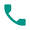## Canadian universities with high acceptance rate

posted on: January 19, 2023

There are some universities that have a very low acceptance rate which means that they admit only a few students annually in their programmes. But there are a lot of universities which do not have extremely low acceptance rates. Students have higher chances of getting into these universities as they are not extremely selective. Here is the list of universities in Canada with a generous acceptance rate-

1. University of Lethbridge

This university has an acceptance rate of 93%. Students with a GPA of three can get into this university. The annual tuition fee here is C\$20,090

1. University of Winnipeg

This university has an acceptance rate of 75%. It has a very affordable tuition fee of C\$13,000 annually.

1. Memorial University of Newfoundland

This university has an acceptance rate of 67%. It has a very affordable tuition fee and the programmes start with the tuition fee as low as C\$6000 a year.

1. Brandon University

This university has an acceptance rate of 47%. If you opt for a three hour credit programme, then the tuition fee will be somewhere around C\$2500. If you opt for a 30 hour credit programme then the tuition fee will be somewhere around C\$17,500.

## Subscribe